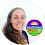Hen Egg Banana Puzzle - Can You solve the last equation ?

Explanation
Equation 1: 20 + 20 + 20 = 60 (Hen = 20)
Equation 2: 20 + 3 + 3 = 26 (Eggs = 3)
Equation 3: 3 + 6 + 6 = 15 (Bananas = 6; Note that there are 6 bananas in one set)
Equation 4: 20 + (3 x 5) = 35 (There are only 5 bananas in this set, so bananas = 5)

1.1.2.2.3.